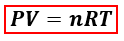# Problem: The combustion of octane, C8H18, proceeds according to the reaction2C8H18(l) + 25O2(g) → 16CO2(g) + 18H2O(l) If 305 mol of octane combusts, what volume of carbon dioxide is produced at 35.0°C and 0.995 atm?

###### FREE Expert Solution
80% (386 ratings)
###### FREE Expert Solution

We are asked to calculate the volume of carbon dioxide (CO2) produced from the combustion of octane (C8H18). We can calculate the volume of the gas using the ideal gas equation:P = pressure, atm
V = volume, L
n = moles, mol
R = gas constant = 0.08206 (L·atm)/(mol·K)
T = temperature, K

In the ideal gas equation, the amount of CO2 in moles is needed but the moles of C8H18 is given instead. We will have to calculate the moles of CO2 produced from CH4 first using the balanced reaction equation and mole to mole comparison.

80% (386 ratings)###### Problem Details

The combustion of octane, C8H18, proceeds according to the reaction

2C8H18(l) + 25O2(g) → 16CO2(g) + 18H2O(l)

If 305 mol of octane combusts, what volume of carbon dioxide is produced at 35.0°C and 0.995 atm?

What scientific concept do you need to know in order to solve this problem?

Our tutors have indicated that to solve this problem you will need to apply the Gas Stoichiometry concept. You can view video lessons to learn Gas Stoichiometry. Or if you need more Gas Stoichiometry practice, you can also practice Gas Stoichiometry practice problems.

What is the difficulty of this problem?

Our tutors rated the difficulty ofThe combustion of octane, C8H18, proceeds according to the r...as medium difficulty.

How long does this problem take to solve?

Our expert Chemistry tutor, Sabrina took 6 minutes and 3 seconds to solve this problem. You can follow their steps in the video explanation above.

What professor is this problem relevant for?

Based on our data, we think this problem is relevant for Professor McCarty's class at NCSU.